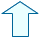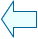TESSELLATING THE PLANE Section: Geometry

1. TESSELLATING THE PLANE. The first way.

This involves finding out which shaped tile can be used to tile a floor. Of course squares can be used and the floors in a house are usually tiled using square tiles.

1.- Move each tile (drag the point inside the tile) and join as many as you can together around point T.

2.- How many squares meet at each vertex? Did you get 4? This is because each interior angle is 90º so we need 4 to cover (tessellate) 360º as

360º=4·90º

 The Init button will restore the initial values and allow you to try other combinations.

2.  TESSELLATING THE PLANE. The second way.
Is it possible to tessellate the plane with hexagons? Try it and see:
1.- How many hexagons meet at each vertex? Can you explain why as we did with the squares.

2.-What does this form of tessellation remind you of? Where can we find it in nature?

 The Init button will restore the initial values.

3. TESSELLATING THE PLANE. The third way?
Try to join the pentagons together to tessellate the plane.

1.- Can you use a formula to explain why it isn't possible?
 Use the rotate_B, rotate_C, rotate_D and rotate_E control buttons to try and join up the sides of the pentagons around T.

4. TESSELLATING THE PLANE. The fourth way

There is only one other type of regular tile that can be used to tessellate the plane, which you can see in the following window:

1.- Move each tile (drag the point inside the shape) and join as many as you can together around point T.

 Use the rotate_B, rotate_C, rotate_D and rotate_E control buttons to join up the sides of the triangles around T.

2.- How many triangles meet at each vertex? Write an explanation for this in your exercise book.Agustín Muñoz NúñezSpanish Ministry of Education. Year 2001